Chapter 2. Making Measurements

# 2.5 Density – Just Another Conversion Factor

### Learning Objectives

By the end of this section, you will be able to:

• Define density and use it as a conversion factor.

Density is a physical property that is defined as a substance’s mass divided by its volume:

density = mass/volume  or d = m/V

Density is usually a measured property of a substance, so its numerical value affects the significant figures in a calculation. Notice that density is defined in terms of two dissimilar units, mass and volume. That means that density overall has derived units, just like velocity. Common units for density include g/mL, g/cm3, g/L, kg/L, and even kg/m3. Densities for some common substances are listed in Table 1 “Densities of Some Common Substances”.

Table 1. Densities of Some Common Substances

Substance Density (g/mL or g/cm3)
water 1.0
gold 19.3
mercury 13.6
air 0.0012
cork 0.22–0.26
aluminum 2.7
iron 7.87

Because of how it is defined, density can act as a conversion factor for switching between units of mass and volume. For example, suppose you have a sample of aluminum that has a volume of 7.88 cm3. How can you determine what mass of aluminum you have without measuring it? You can use the volume to calculate it. If you multiply the given volume by the known density (from Table 1 “Densities of Some Common Substances”), the volume units will cancel and leave you with mass units, telling you the mass of the sample:

7.88 cm3 × 2.7 g/cm= 21 g of aluminum

where we have limited our answer to two significant figures.

### Example 1

What is the mass of 44.6 mL of mercury?

Solution

Use the density from Table 1 “Densities of Some Common Substances” as a conversion factor to go from volume to mass:

44.6 mL × 13.6 g/mL = 607 g

The mass of the mercury is 607 g.

Test Yourself

What is the mass of 25.0 cm3 of iron?

197 g

Density can also be used as a conversion factor to convert mass to volume—but care must be taken. We have already demonstrated that the number that goes with density normally goes in the numerator when density is written as a fraction. Take the density of gold, for example: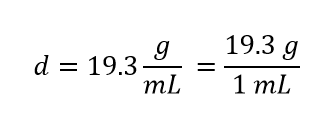Although this was not previously pointed out, it can be assumed that there is a 1 in the denominator:

That is, the density value tells us that we have 19.3 grams for every 1 milliliter of volume, and the 1 is an exact number. When we want to use density to convert from mass to volume, the numerator and denominator of density need to be switched—that is, we must take the reciprocal of the density. In so doing, we move not only the units but also the numbers: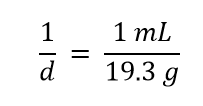This reciprocal density is still a useful conversion factor, but now the mass unit will cancel and the volume unit will be introduced. Thus, if we want to know the volume of 45.9 g of gold, we would set up the conversion as follows: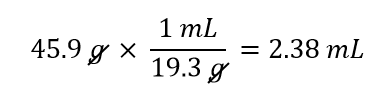Note how the mass units cancel, leaving the volume unit, which is what we’re looking for.

### Example 2

A cork stopper from a bottle of wine has a mass of 3.78 g. If the density of cork is 0.22 g/cm3, what is the volume of the cork?

Solution

To use density as a conversion factor, we need to take the reciprocal so that the mass unit of density is in the denominator. Taking the reciprocal, we find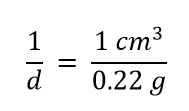We can use this expression as the conversion factor. So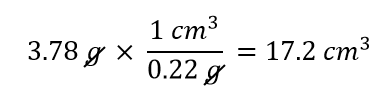Then, taking significant figures into consideration, since the density only has two significant figures, the final answer is 17 cm3.

Test Yourself

What is the volume of 3.78 g of gold?

0.196 cm3

### Example 3

If a 5.00 g sample has a density of 2.50 g/mL, what volume does it occupy?

Solution

Look at the density value as a “conversion factor” (2.50 g/mL) and arrange it so it CANCELS what you already know. Thus, in this case we must invert it.

Multiply and cancel units…

$5.00\;\rule[0.75ex]{1.0em}{0.1ex}\hspace{-1.0em}\text{g} \times \frac{1\;\text{mL}}{2.50\;\rule[0.25ex]{0.5em}{0.1ex}\hspace{-0.5em}\text{g}} = 2.00\;\text{mL}$

Test Yourself

a)   Isopropyl alcohol has a density of 0.785 g/mL.
What volume should be measured to obtain 10.0 g of the liquid?

b)   A cube of metal has a mass of 1.45 kg. It is placed in 200.0 mL of water, and the water level rises to 742.1 mL. What is the density of the metal?

a) 12.7 mL          b) 2.67 g/mL

Care must be used with density as a conversion factor. Make sure the mass units are the same, or the volume units are the same, before using density to convert to a different unit. Often, the unit of the given quantity must be first converted to the appropriate unit before applying density as a conversion factor.

## Key Concepts and Summary

Density relates a substance’s mass and volume. Density can be used to calculate volume from a given mass or mass from a given volume.

### Exercises

1.  Give at least three possible units for density.

2.  A sample of iron has a volume of 48.2 cm3. What is its mass?

3.  The volume of hydrogen used by the Hindenburg, the German airship that exploded in New Jersey in 1937, was 2.000 × 108 L. If hydrogen gas has a density of 0.0899 g/L, what mass of hydrogen was used by the airship?

4.  A typical engagement ring has 0.77 cm3 of gold. What mass of gold is present?

5.  What is the volume of 100.0 g of lead if lead has a density of 11.34 g/cm3?

6.  What is the volume in liters of 222 g of neon if neon has a density of 0.900 g/L?

7.  Which has the greater volume, 100.0 g of iron (d = 7.87 g/cm3) or 75.0 g of gold (d = 19.3 g/cm3)?

1.  g/mL, g/L, and kg/L (answers will vary)

2.  379 g

3.  1.80 × 107 g

4.  15 g

5.  8.818 cm3

6.  247 L

7.  The 100.0 g of iron has the greater volume.